Publicité

# Wavelet estimation for a multidimensional acoustic or elastic earth

29 Oct 2015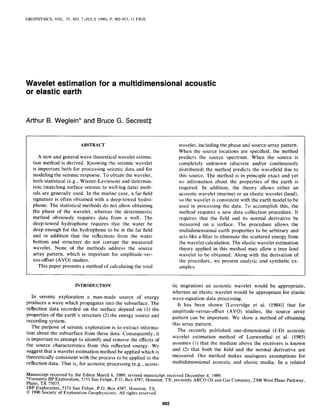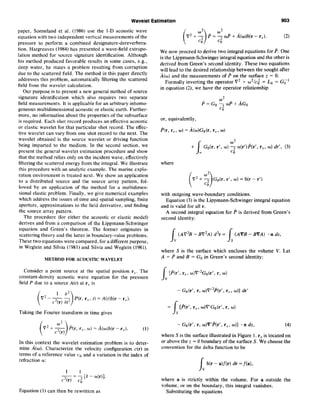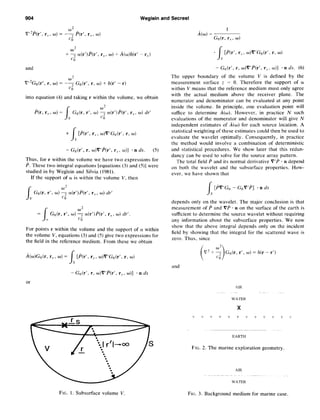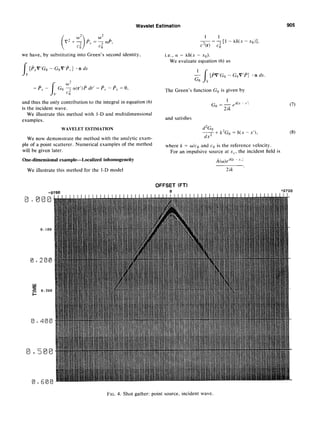Publicité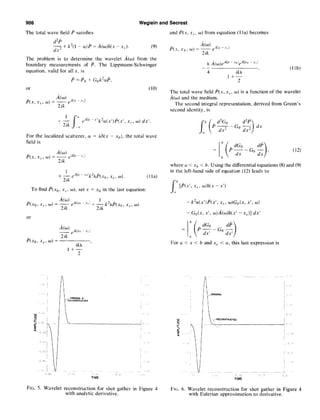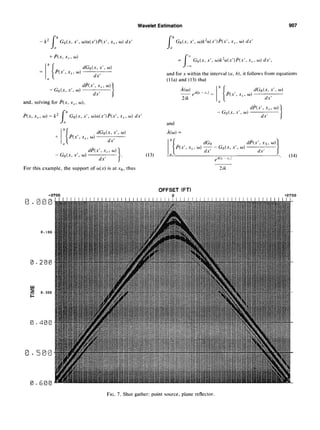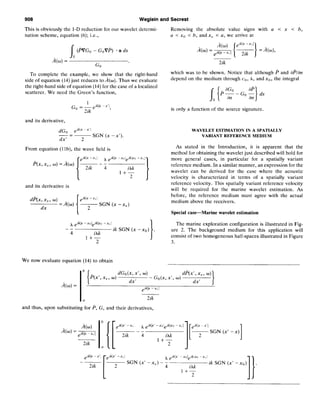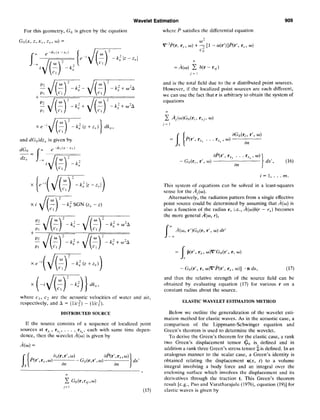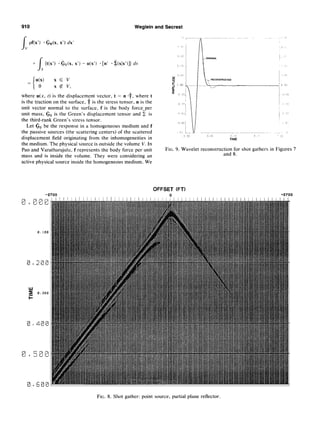Publicité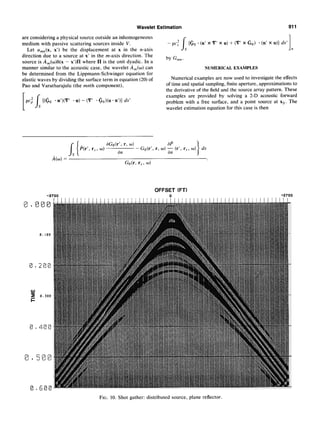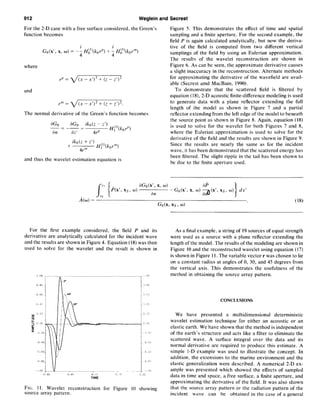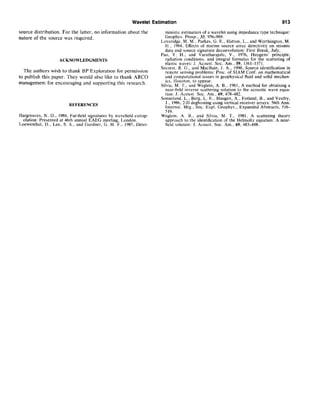Prochain SlideShare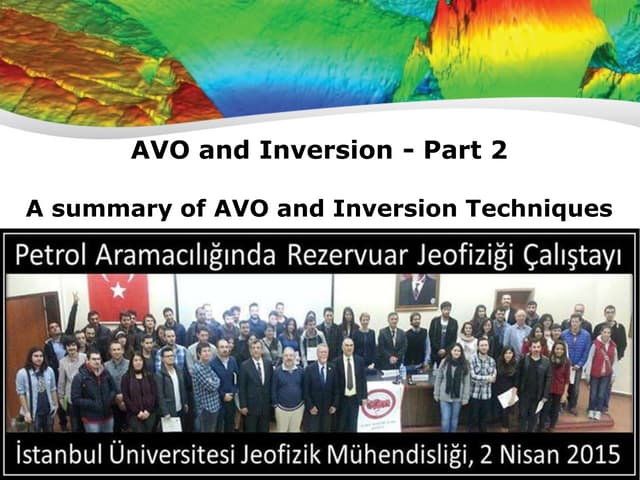Reservoir Geophysics : Brian Russell Lecture 2
Chargement dans ... 3
1 sur 12
Publicité

### Wavelet estimation for a multidimensional acoustic or elastic earth

1. GEOPHYSICS, VOL. 55, NO. 7 (JULY 1990); P. 902-913, I1 FlGS Wavelet estimation for a multidimensional acoustic or elastic earth Arthur B. Weglein* and Bruce G. Secrest$ABSTRACT A new and general wave theoretical wavelet estima- tion method is derived. Knowing the seismic wavelet is important both for processingseismic data and for modeling the seismicresponse.To obtain the wavelet, both statistical (e.g., Wiener-Levinson) and determin- istic (matching surface seismicto well-log data) meth- ods are generally used. In the marine case, a far-field signature is often obtained with a deep-towed hydro- phone. The statistical methodsdo not allow obtaining the phase of the wavelet, whereas the deterministic method obviously requires data from a well. The deep-towed hydrophone requires that the water be deep enough for the hydrophone to be in the far field and in addition that the reflections from the water bottom and structure do not corrupt the measured wavelet. None of the methods address the source array pattern, which is important for amplitude-ver- sus-offset(AVO) studies. This paper presentsa methodof calculating the total wavelet, including the phaseand source-arraypattern. When the source locations are specified, the method predicts the source spectrum. When the source is completely unknown (discrete and/or continuously distributed) the method predicts the wavefield due to this source. The method is in principle exact and yet no information about the properties of the earth is required. In addition, the theory allows either an acousticwavelet (marine) or an elastic wavelet (land), sothe wavelet is consistentwith the earth model to be used in processingthe data. To accomplish this, the method requires a new data collection procedure. It requires that the field and its normal derivative be measured on a surface. The procedure allows the multidimensional earth properties to be arbitrary and acts like a filter to eliminate the scatteredenergy from the wavelet calculation. The elastic wavelet estimation theory applied in this method may allow a true land wavelet to be obtained. Along with the derivation of the procedure, we present analytic and synthetic ex- amples. INTRODUCTION tic migration) an acoustic wavelet would be appropriate, I . . . whereasan elastic wavelet would be appropriate for elastic wave-equation data processing.In seismic exploration a man-made source ot energy producesa wave which propagatesinto the subsurface.The reflection data recorded on the surface depend on (1) the properties of the earth’s structure (2) the energy sourceand recording system. The purpose of seismic exploration is to extract informa- tion about the subsurfacefrom these data. Consequently, it is important to attempt to identify and remove the effectsof the source characteristics from this reflected energy. We suggestthat a wavelet estimation method be applied which is theoretically consistentwith the processto be applied to the reflection data. That is, for acousticprocessing(e.g., acous- It has been shown [Loveridge et al. (1984)] that for amplitude-versus-offset (AVO) studies, the source array pattern can be important. We show a method of obtaining this array pattern. The recently published one-dimensional (1-D) acoustic wavelet estimation method of Loewenthal et al. (1985) assumes(1) that the medium above the receivers is known and (2) that both the field and the normal derivative are measured. Our method makes analogous assumptions for multidimensional acoustic and elastic media. In a related Manuscript received by the Editor March 6, 1989;revised manuscriptreceived December4, 1989. *Formerly BP Exploration, 5151SanFelipe, P.O. Box 4587,Houston,TX; presentlyARC0 Oil and GasCompany, 2300West PlanoParkway, Plano, TX 75075. SBPExploration, 5151San Felipe, P.O. Box 4587, Houston, TX. 0 1990Society of Exploration Geophysicists.All rightsreserved. 2. Wavelet Estimation 903 paper, Sonneland et al. (1986) use the 1-D acoustic wave “2 w2 equationwith two independentvertical measurementsof the pressure to perform a combined designature-dereverbera- ( )V2 + 2 P = 2 09 + A(w)s(r - r,). (2) tion. Hargreaves(1984) has presenteda wave-field extrapo- lation method for source signatureidentification. Although his method producedfavorable results in some cases,e.g., deep water, he statesa problem resulting from corruption due to the scatteredfield. The methodin this paperdirectly addressesthis problem, automatically filtering the scattered field from the wavelet calculation. Our purposeis to presenta new generalmethodof source signature identification which also requires two separate field measurements.It is applicablefor an arbitrary inhomo- geneousmultidimensional acousticor elasticearth. Further- more, no information about the propertiesof the subsurface is required. Each shotrecordproducesan effectiveacoustic or elastic wavelet for that particular shotrecord. The effec- tive wavelet can vary from one shotrecordto the next. The wavelet obtained is the source wavelet or driving function being imparted to the medium. In the second section, we We now proceedto derive two integral equationsfor p. One isthe Lippmann-Schwingerintegral equationandthe otheris derived from Green’s secondidentity. These two equations will leadto the desiredrelationshipbetween the soughtafter A(w) and the measurementsof p on the surfacez = 0. Formally inverting the operatorV2 + w2/ci = La = Cc’ in equation (2), we have the operatorrelationship lo* _ _ P=GoTaP+AGo co or, equivalently, P(r, rs9 01 = AblG~(r, rs, 4 co= + presentthe generalwavelet estimation procedureand show G&, r’, w) _r a(r’)P(r’, rs, O) dr’, (3) CO that the methodrelies only on the incident wave, effectively filteringthe scatteredenergyfrom the integral. We illustrate where this procedurewith an analytic example. The marine explo- ration environment is treated next. We showan application to a distributed source and the source array pattern, fol- G&, r’, w) = S(r - r’) lowed by an application of the method for a multidimen- sionalelasticproblem. Finally, we give numerical examples with outgoingwave-boundary conditions. which addressthe issuesof time and spatialsampling,finite Equation (3) is the Lippmann-Schwingerintegral equation aperture, approximationsto the field derivative, and finding and is valid for all r. the sourcearray pattern. A secondintegral equation for P is derived from Green’s The procedure (for either the acoustic or elastic model) secondidentity: derivesand from a comparisonof the Lippmann-Schwinger equation and Green’s theorem. The former originates in scatteringtheory and the latter in boundary-value problems. I (AV2B - BV’A) d3r = (AVB - BVA) . n ds, Thesetwo equationswere compared,for a differentpurpose, V I s in Weglein and Silvia (1981) and Silvia and Weglein (1981). where S is the surface which enclosesthe volume V. Let METHODFORACOUSTICWAVELET A = P and B = Go in Green’s secondidentity: Consider a point source at the spatial position r,. The constant-density acoustic wave equation for the pressure s[h-‘, rs, w)V’*Go(r’, r, W) V field P due to a sourceA(t) at r,?is (y&g) - Go(r’, r, w)Vt2p(r’, rs, w)] dr’ P(r, rsr t) = A(t)G(r - r,). Taking the Fourier transform in time gives = [f%‘, rs, w)V’Go(r’, r, w) iJJ2_ ( >V2 + - P(r, rs, w) = A(w)s(r - r,). (1) - Go(r’, r, o)V’P(r’, rs, w)] - n ds, (4) c’(r) whereS is the surfaceillustratedin Figure 1. rs is locatedon In this context the wavelet estimation problem is to deter- or abovethe z = 0 boundaryof the surfaceS. We choosethe mine A(w). Characterize the velocity configuration c(r) in convention for the delta function to be termsof a referencevalue cn and a variation in the index of refraction (Y: / 6(r - a)f(r) dr =f(i), 1 I V - = - [I - ff(r)]. c’(rj ci where P is strictly within the volume. For amoutside the volume, or on the boundary, this integral vanishes. Equation (1) can then be rewritten as Substitutingthe equations 3. 904 Weglein and Secrest V’ ‘P(rl, r,5 , Lo2 0) = -1 P(r’, r,s, 0) (‘0 + 1 a(r’)P(r’, r,, , 0) + A(w)S(r’ - r,) co and 2 Vf2Go(r’, r, w) = -q Go(r’, r, w) + S(r’ - r) co into equation (4) and taking r within the volume, we obtain s 2 P(r, rs, 0) = Go(r, r’, co) ” a(r’)B(r’, r,, , o) dr’ v co’ + [%-‘, rs, o)V’Go(r’, r, 01 - G”(r’, r, w)V’P(r’, rr, o)] *n ds. (5) Thus, for r within the volume we have two expressions for p. These two integral equations [equations (3) and (5)] were studied in by Weglein and Silvia (1981). If the support of a is within the volume V, then 7 Go(r, r’, w) ” a(r’)&‘, rs, O) dr’ c; I w2 = Go(r, r’, co) 1 a(r’)P(r’, r,, , w) dr’. m C6 For points r within the volume and the support of (Ywithin the volume V, equations (3) and (5) give two expressions for the field in the reference medium. From these we obtain - Go(r’, r, o)V’&, r,r, w)] . n ds or FIG. 1. Subsurface volume V. I A(w) = Go(r, rs, co) *I [I%-‘, r,, w)V’Go(r’. r, w) 5 - COW. r, w)V’p(r’, r,, co)] *n ds. (6) The upper boundary of the volume V is defined by the measurement surface z = 0. Therefore the support of (Y within V means that the reference medium must only agree with the actual medium above the receiver plane. The numerator and denominator can be evaluated at any point inside the volume. In principle, one evaluation point will suffice to determine /i(w). However, in practice N such evaluations of the numerator and denominator will give N independent estimates of A(w) for each source location. A statistical weighting of these estimates could then be used to evaluate the wavelet optimally. Consequently, in practice the method would involve a combination of deterministic and statistical procedures. We show later that this redun- dancy can be used to solve for the source array pattern. The total field P and its normal derivative V’P . n depend on both the wavelet and the subsurface properties. How- ever. we have shown that [f%‘Go - GoV’P] - n ds depends only on the wavelet. The major conclusion is that measurement of ls and VP . n on the surface of the earth is sufficient to determine the source wavelet without requiring any information about the subsurface properties. We now show that the above integral depends only on the incident field by showing that the integral for the scattered wave is zero. Thus, since Go(r, r’, w) = 6(r - r’) and EARTH FIG. 2. The marine exploration geometry. AIR WATER Fto. 3. Background medium for marine case. 4. Wavelet Estimation 905 Gj Cci we have, by substituting into Green’s second identity, / [P,V’G,, - GuV’P,] - n ds s I W2 = p, - Go 7 a(r’)P dr’ = p,, - P, = 0, V C’iJ and thus the only contribution to the integral in equation (6) is the incident wave. We illustrate this method with 1-D and multidimensional examples. WAVELET ESTIMATION We now demonstrate the method with the analytic exam- ple of a point scatterer. Numerical examples of the method will be given later. One-dimensional example-Localized inhomogeneity We illustrate this method for the I-D model I - = _!-[l - h6(x -X0)], c’(r) co’ i.e., (Y= hS(x - x0). We evaluate equation (6) as [pV’GO - GOV’P] - n ds. The Green’s function Go is given by I Go = _ eikix 1’1 2ik and satisfies d2Go dx2 + k2Go = 6(x -x’), where k = o/co and cg is the reference velocity. For an impulsive source at x,, the incident field is 2ik . (8) OFFSET (FT) FIG. 4. Shot gather: point source, incident wave. 5. 906 The total wave field P satisfies Weglein and Secrest d2P and P(.u, x,, O) from equation (1la) becomes ---Q + k2(1 - oi)P = A(o)S(x- - X,). (9) The problem is to determine the wavelet A(w) from the boundary measurements of P. The Lippmann-Schwinger equation, valid for all x, is or 1 +- 2ik (~‘k’“~.“ik’a(x’)P(x’. x,~, w) dx’. For the localized scatterer, (Y= hF(x - x0), the total wave field is To find P(n-,,,x,, w), set x = x0 in the last equation: or &I, x,s,w) = 2ik ikh ’ l+y (1la) A A(w)P iklx-roieikl.ro -xti -- 4 ikX I+7 (I lb) The total wave field p(x, x,, w) is a function of the wavelet A(w) and the medium. The secondintegral representation, derived from Green’s secondidentity, is where a < x,) < h. Using the differential equations(8) and (9) in the left-hand side of equation (12) leads to d'G,, d"P p----dx2 Go -dx2 dx Ih[P(x’, x,, , w)S(x - x’) 0 - k2a(x’)P(x’, x,~, o)Go(x, x’, co) - Go(x, x’, w)&o)S(x’ - x,~)]dx' b I(_dGo di, 1 pdx’-G,dx’ u For CI< x < b and x,~< a, this last expressionis = 1; (+G,, g), (12) FIG. 5. Wavelet reconstruction for shot gather in Figure 4 with analytic derivative. FK. 6. Wavelet reconstruction for shot gather in Figure 4 with Eulerian approximation to derivative. 6. Wavelet Estimation 907 -k= b Go(x, x’, w)a(x’)li(x’, xs, w) dx’ b II &‘, x, >0) dGo(x, x’, 0) dx' 0 - Go(x,x’, 01 dP(x’, x, >01 dx' Iand, solving for P(x, xS, o), p(x, xs, CO)= k= b G,,(x, x’, w)a(x’)P(x’, xs, w) dx’ b Ii dGo(x, x’, w) + &‘, x, 10) dx’ u - Gotx, x’, w) For this example, the support of a(x) is at x0, thus (13) I b Go(x, x’, w)k=a(x’)P(x’, x,, o) dx’ a s ^a = Go(x, x’, o)k*a(x’)P(x’, x,~. w) dx’, --53 and for x within the interval (a, b), it follows from equations (lla) and (13) that P(x’, x,, WI dGo(x, x’, co) dx’ - Go(x, I’, w) d&‘, x, , w) dx’ and$0) = dGo P(x’, x,, w) dx’ - Go(x, x’, w) drj(x’, x5, w) dx’ /gklx x, j (14) 2ik OFFSET (FT) FIG. 7. Shot gather: point source, plane reflector.
7. 908 Weglein and Secrest This is obviously the 1-D reduction for our wavelet detcrmi- nation scheme, equation (6); i.e., Removing the absolute value signs with a < x < b, u i x0 < b, andx, < a, we arrive at I @Go - GoVf’) *n ds sk(o) = Cl, To complete the example, WC show that the right-hand side of equation (14) just reduces to k(w). Thus we evaluate the right-hand side of equation (14) for the case of a localized scatterer. We need the Green’s function. and its derivative, dGo &V mx’i -= dx’ ~ SGN (x -x’). 2 From equation (lib), the wave field is c”l” --x,1 and its derivative is SGN (x -x,) ik SGN (x -x,,) We now evaluate equation (14) to obtain 2ik which was to be shown. Notice that although P and #Iian depend on the medium through co, h, and x,,, the integral is only a function of the source signature. WAVELET ESTIMATION IN A SPATIALLY VARIANT REFERENCE MEDIUM As stated in the Introduction, it is apparent that the method for obtaining the wavelet just described will hold for more general cases, in particular for a spatially variant reference medium. In a similar manner, an expression for the wavelet can be derived for the case where the acoustic velocity is characterized in terms of a spatially variant reference velocity. This spatially variant reference velocity will be required for the marine wavelet estimation. As before, the reference medium must agree with the actual medium above the receivers. Special case-Marine wavelet estimation The marine exploration configuration is illustrated in Fig- ure 2. The background medium for this application will consist of two homogeneous half-spaces illustrated in Figure 3. b &‘, xs7 WI dGo(x, x’, ~1 d&’ ,x.7, ~1 k(w) = dx’ - Go(x, x’, w) dx’ p+ --x.,j a 2ik and thus, upon substituting for P, G, and their derivatives, 2ik r eikb’ --xs h eiklY’ -rii~elk~il --x5 / eikl-x’/ ~-- 2ik 4 ~ SGN (x’ -x) 2 -~ 2ik ~ SGN (x’ -x,~) - ; 2 iti ik SGN (x’ -x0) . l+2 II
8. Wavelet Estimation 909 For this geometry, G,, is given by the equation and dGo/dz,7is given by dGo -= dz, I_: ii& - k,"SGN (z, - z) Si(J(;)2-k;(z+z,y)) x (-id(,)I-k:)} dk,, where ct , c2 are the acoustic velocities of water and air, respectively, and A = (l/c:) - (l/c;). DISTRIBUTED SOURCE If the source consists of a sequence of localized point sources at r,v,, rs,, . . _, r7 , each with same time depen- dence, then the wavelet A(‘;) is given by k(w) = &-‘,r,,w) %(r,r’,co) @r’, r,, ~1 an -G~(r,r’,w) ds’ an ? (15) where P satisfies the differential equation V”P(r, I-~, w) + 1 [l - a(r’)]P(r’, r,T, w) Cl) =,4(w) i 6(r - r.5j) ;=I and is th-e total fieldsdue tomthe ;r distributed point sourcesi However, if the localized point sources are each different, we can use the fact that r is arbitrary to obtain the system of equations J&h, r’, ~1 = B(r’, r,, . . . ry,) , 0) an dP(r’, r,s, . . . rs, , 0) - GA-,, r’, 0) an ds’, 06) i=l,...m. This system of equations can be solved in a least-squares sense for the k,(w). Alternatively, the radiation pattern from a single effective point source could be determined by assuming that k(o) is also a function of the radius r, i.e., A(w)6(r - r,?)becomes the more general k(w, r), I co k(o, r’)G,,(r, r’, co)dr’ --(c ZZ I fib’, r,, w)V’G&‘, r, w) s - G,,(r’, r, o)V’P(r’, rs, co)] *n ds, (17) and thus the relative strength of the source field can be obtained by evaluating equation (17) for various r on a constant radius about the source. ELASTIC WAVELET ESTIMATION METHOD Below WC outline the generalization of the wavelet esti- mation method for elastic waves. As in the acoustic case, a comparison of the Lippmann-Schwinger equation and Green’s theorem is used to determine the wavelet. To derive the Green’s theorem for the elastic case, a rank two Green’s displacement tensor so is_ defined and in addition a rank three Green’s stress tensor $is defined. In an analogous manner to the scalar case, a Green’s identity is obtained relating the displacement u(x, t) to a volume integral involving a body force and an integral over the enclosing surface which involves the displacement and its derivatives through the traction t. This Green’s theorem result [e.g., Pao and Varatharajulu (1976), equation (19)] for elastic waves is given by 9. 910 Weglein and Secrest / pf(x’) * i&(x, x’) dx’ V + I {t(x’) *&(x, x’) - u(x’) *[n’ -$(x1x’)]} cf.5 s 44 XEV zz 0 x @ v, where u(x, t) is the displacement vector, t = n .‘f, where t is the traction on the surface, T is the stress tensor, n is the unit vector normal to the surface, f is the body force=per unit mass, CO is the Green’s displacement tensor and 7 is the third-rank Green’s stress tensor. Let GO be the response in a homogeneous medium and f the passive sources (the scattering centers) of the scattered displacement field originating from the inhomogeneities in the medium. The physical source is outside the volume V. In Pao and Varatharajulu, f represents the body force per unit mass and is inside the volume. They were considering an active physical source inside the homogeneous medium. We FIG. 9. Wavelet reconstruction for shot gathers in Figures 7 and 8. OFFSET (FT) -2700 +2700 0 0000 llllllllllllllllllllllIllllll,PilIIllIllllllllllllllllllIIlll 0.100 0.200 ; 0.300 0.400 0 - 500 0.600 FIG. 8. Shot gather: point source, partial plane reflector.
10. Wavelet Estimation 911 are consideringa physical sourceoutsidean inhomogeneous medium with passive scattering sourcesinside V. Let umn(x, x’) be the displacement at x in the n-axis direction due to a source at x’ in the m-axis direction. The sourceis A,(w)s(x - x’)II where II is the unit dyadic. In a manner similar to the acoustic case, the wavelet A,(o) can be determined from the Lippmann-Schwinger equation for elastic waves by dividing the surfaceterm in equation (20) of Pao and Varatharajulu (the mnth component), {(Go - n’)(V’ *u) - (V’ *(&)(u *n’)} ds’ - PCS / {& - (n’ x V’ x u) + (V’ x Go) - (n’ x u)} ds’ s n by G,,. NUMERICAL EXAMPLES Numerical examplesare now usedto investigatethe effects of time and spatialsampling,finite aperture,approximationsto the derivative of the field and the sourcearray pattern. These examples are provided by solving a 2-D acoustic forward problem with a free surface, and a point source at xs. The wavelet estimationequationfor this caseis then dG~(r', r, ~1 aP &-‘, r, , 0) - Gob’, r, w); (r’, rs, 0) ds an Go@,rs, ~1 OFFSET (FT) -2700 +2700 0 m000 Illllllllllllllllllllllllllll19llllllllllllllllllllllllllllll 0. I00 0.200 0.300 F 0.400 0.500 0.600 FIG. 10. Shot gather: distributed source, plane reflector.
11. Wavelet Estimation 913 source distribution. For the latter, no information about the ministicestimationof a wavelet usingimpedancetype technique: nature of the source was required. Geophys. Prosp., 33, 956-969. Loveridge. M. M., Parkes, G. E., Hatton, L., and Worthington, M. H.. 1984. Effects of marine source array directivitv on seismic data and source signature deconvolutionl First Breik, July, Pao, Y. H., and Varatharajulu, V., 1976, Huygens’ principle, ACKNOWLEDGMENTS radiation conditions, and integral formulas for the scattering of elastic waves: J. Acoust. Sot. Am., 59, 1361-1371. The authors wish to thank BP Exploration for permission to publish this paper. They would also like to thank ARC0 management for encouraging and supporting this research. Secrest, B. G.? and MacBain, J. A.. 1990, Source identification in remote sensmg problems: Proc. of SIAM Conf. on mathematical and computational issues in geophysical fluid and solid mechan- its, Houston, to appear. Silvia, M. T.. and Weglein, A. B., 1981, A method for obtaining a near-field inverse scattermg solution to the acoustic wave equa- tion: J. Acoust. Sot. Am., 69, 478-482. REFERENCES Sonneland. L., Berg, L. E., Haugen, A., Fotland, B., and Vestby, J., 1986, 2-D deghosting using vertical receiver arrays: 56th Ann. Internat. Mtg.. Sot. Expl. Geophys., Expanded Abstracts, 516- 519 Hargreaves, N. D., 1984, Far-field signatures by wavefield extrap- elation: Presented at 46th annual EAEG meeting, London. Loewenthal, D., Lee, S. S., and Gardner, G. H. F., 1985, Deter- I *“-Weglem, A. B., and Silvia, M. T., 1981, A scattering theory approach to the identification of the Helmoltz equation: A near- field solution: J. Acoust. Sot. Am., 69, 483-488.
Publicité